# 又来了！10分钟实现微信 "炸屎"大作战

27,291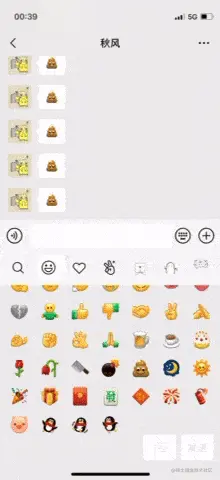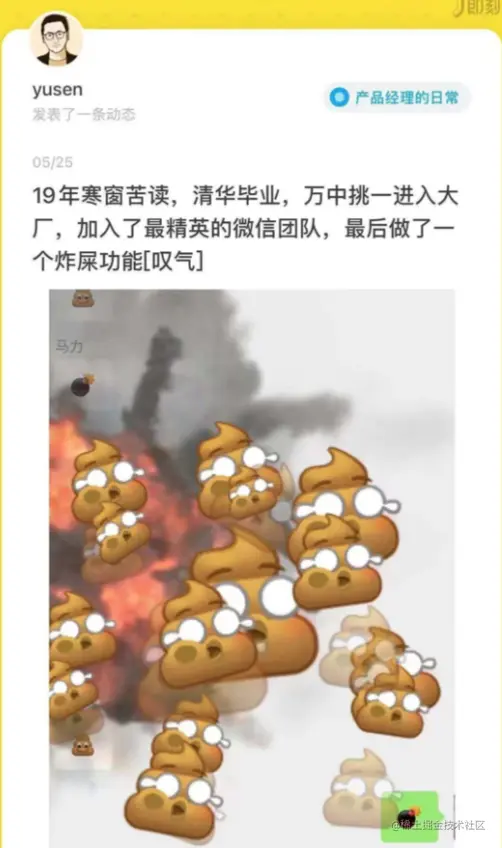example.qiufeng.blue/wechat-fece…

Github地址：

github.com/hua1995116/…

1.丢炸弹

2.炸弹爆炸

3.粑粑被炸开

4.所有人震动

## 具体实现

### 1.丢炸弹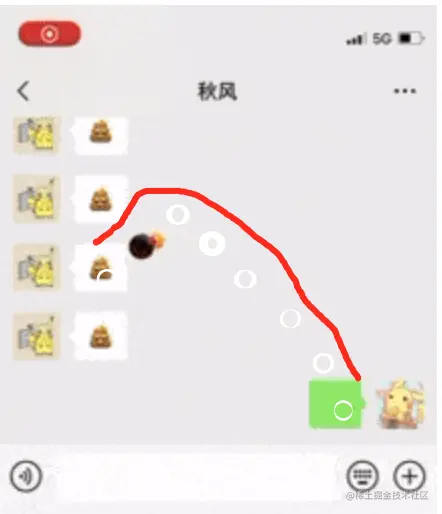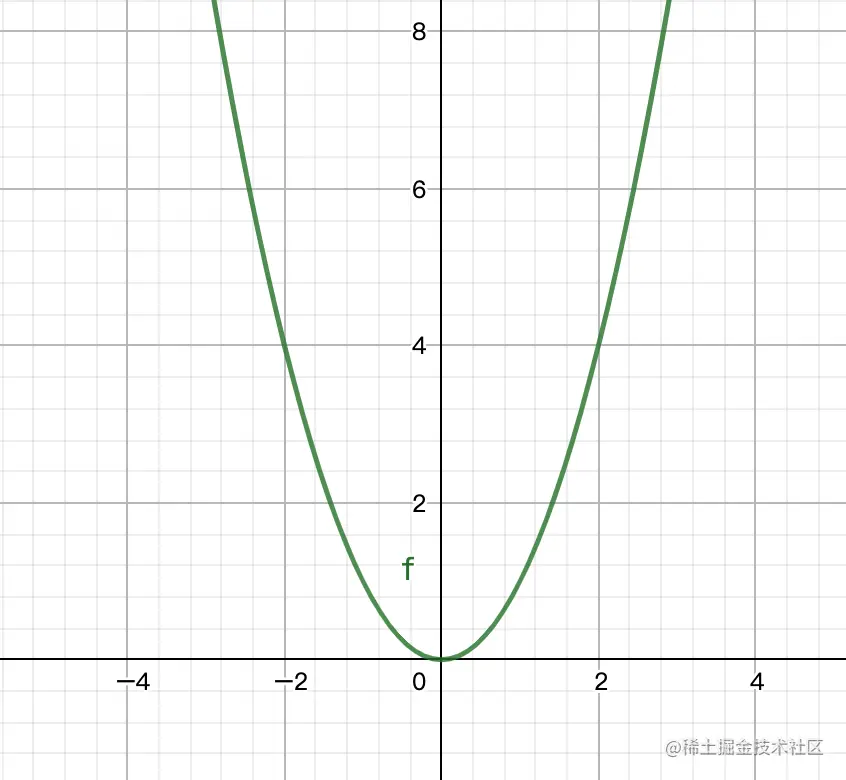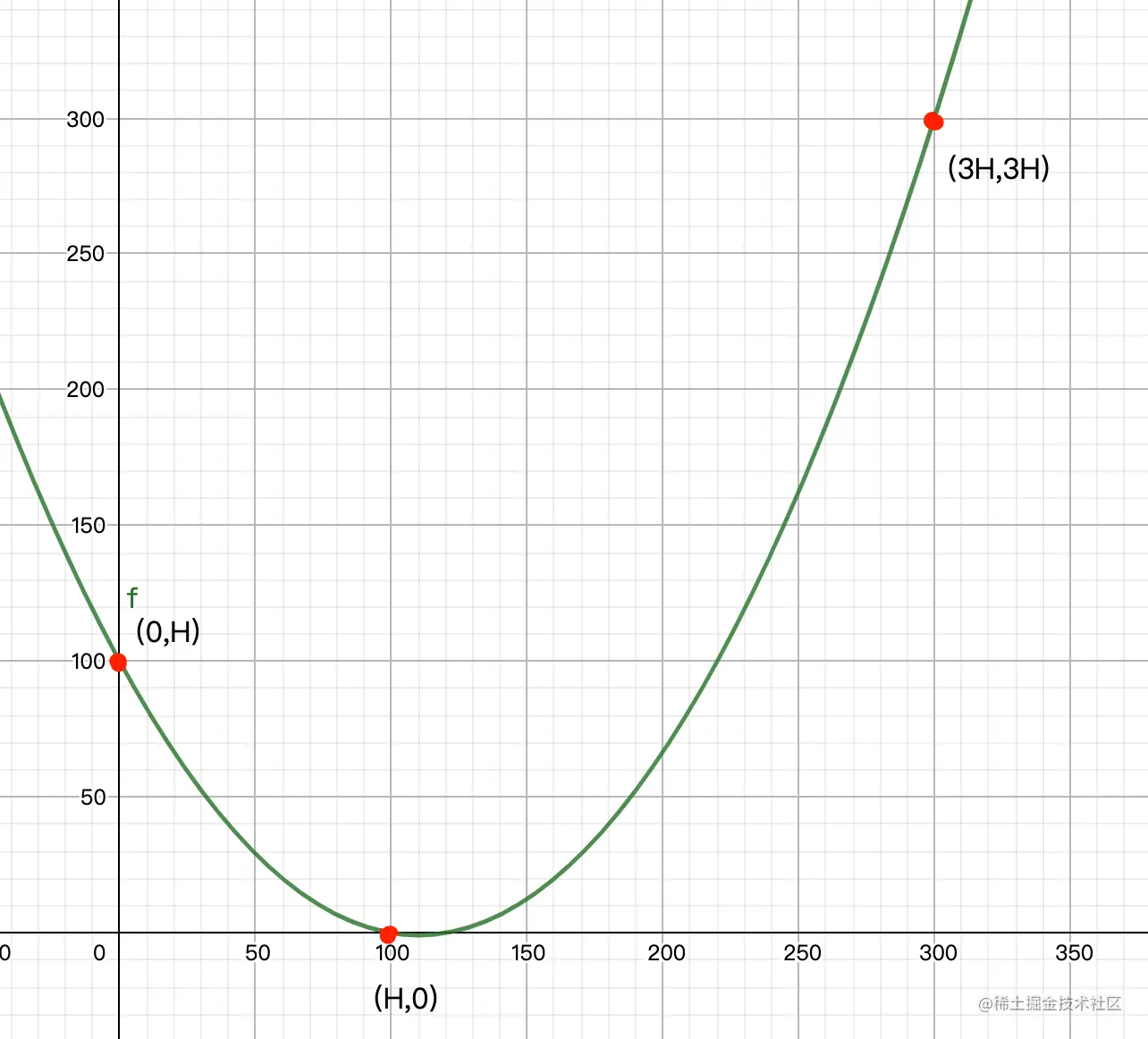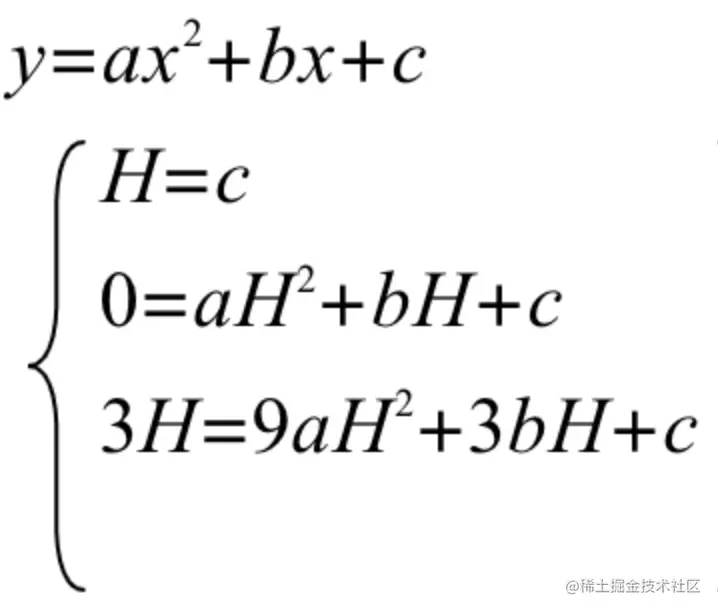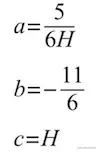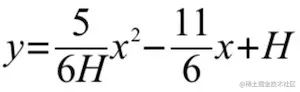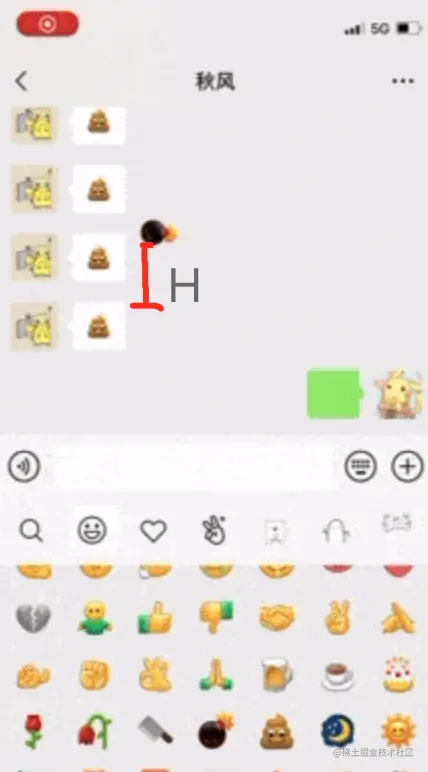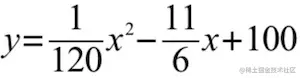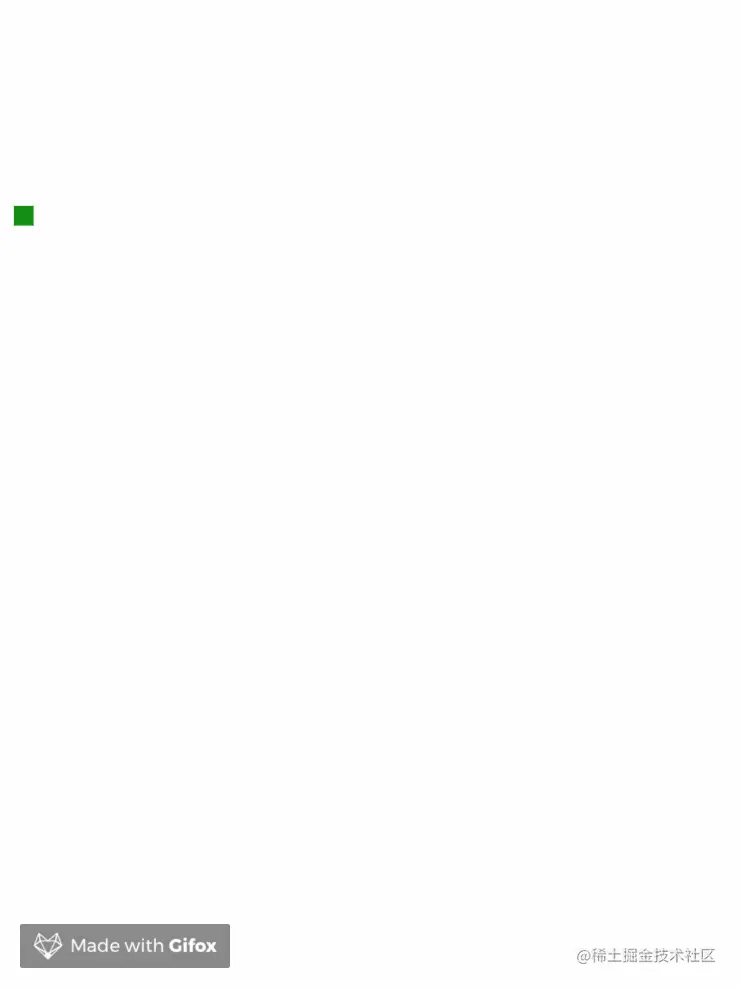var coords = { x: 300 };  // 起始点 为 x = 300
var tween = new TWEEN.Tween(coords)
.to({ x: 0  }, 1000) // 终点为 x = 0, 并且这个动作将在1秒内完成
.easing(TWEEN.Easing.Linear.None)	// 匀速


tween.onUpdate(function() {
var x = coords.x;
var y = 1/120 * x * x - 11/6 * x + 100;
box.style.setProperty('transform', 'translate(' + x + 'px, ' + y + 'px)');
})


1.将方块换成炸弹然，炸弹的形状很简单，我们可以通过 ps 将它从图层中抠出来。

2.修改它运动时候的角度。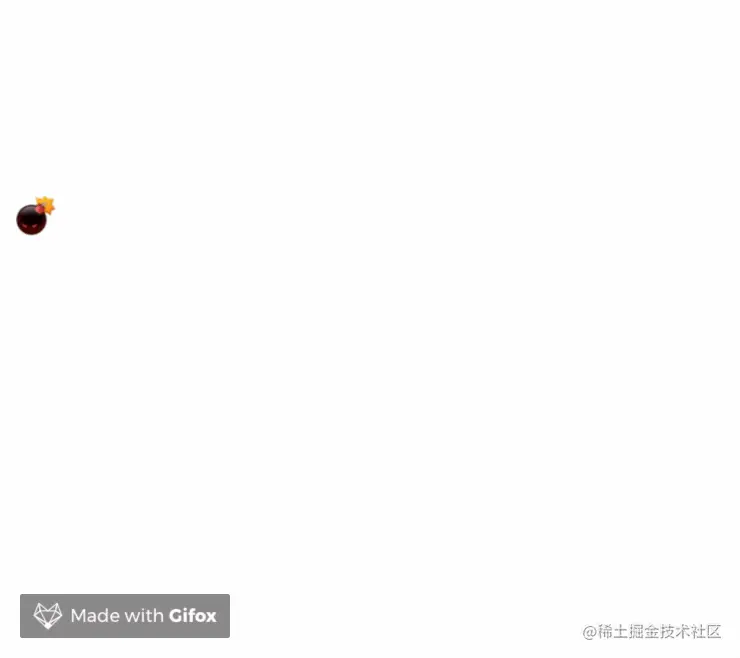### 2.炸弹爆炸

Lottie是一个库，可以解析使用AE制作的动画（需要用bodymovin导出为json格式），支持web、ios、android和react native。在web侧，lottie-web库可以解析导出的动画json文件，并将其以svg或者canvas的方式将动画绘制到我们页面中。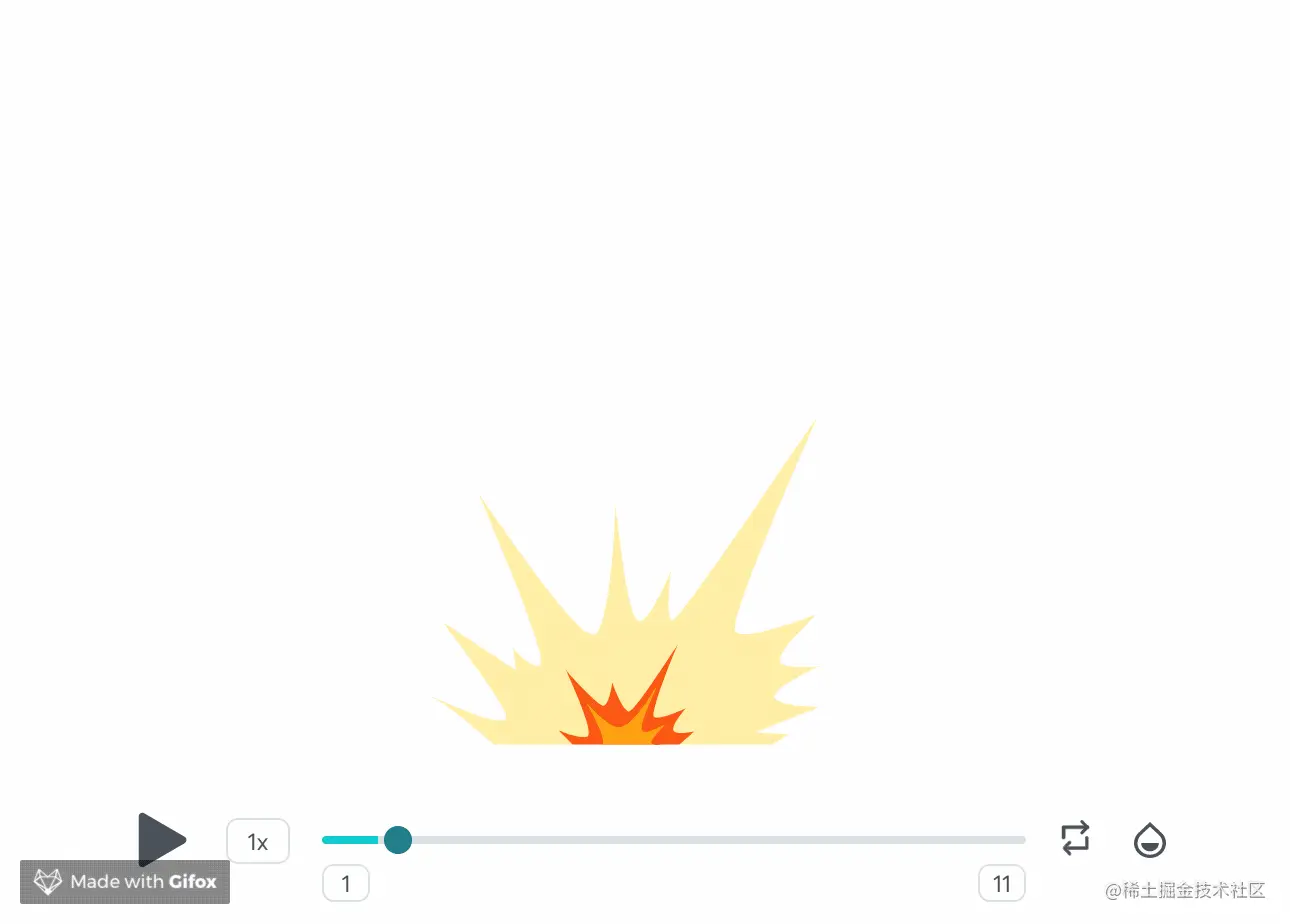<script src="https://cdn.bootcdn.net/ajax/libs/lottie-web/5.7.8/lottie.min.js"></script>
<body>
<div class="bodymovin"></div>
<script>
container: document.querySelector('.bodymovin'), // 要包含该动画的dom元素
renderer: 'svg', // 渲染方式，svg、canvas、html（轻量版仅svg渲染）
loop: true, // 是否循环播放
autoplay: true, // 是否自动播放
path: './bomb.json', // 动画json文件路径
});
</script>


tween.onComplete(function () {
// 写爆炸动画
})### 3.粑粑被炸开

#### 3.1形状.feces {
position: absolute;
background-image: url(./feces.png);
background-size: 100%;
background-position: center;
background-repeat: no-repeat;
width: 80px;
height: 80px;
transform-origin: center;
}

// 创建一个粑粑元素
function createfeces(scale = 1) {
const fece = document.createElement('div');
fece.className = 'feces';
// 由于粑粑有大有小，有方向，因此预留了值。
const symbol = Math.random() > 0.5 ? 1 : -1;
fece.style.transform = scale(${scale * 1.1}) rotate(${symbol * 20 * Math.random()}deg)
return fece;
}


#### 3.2位置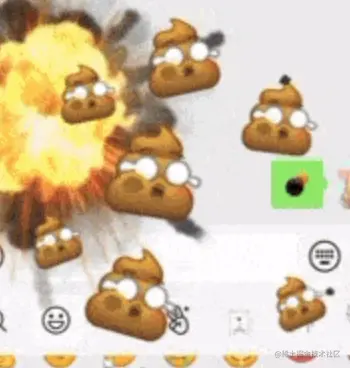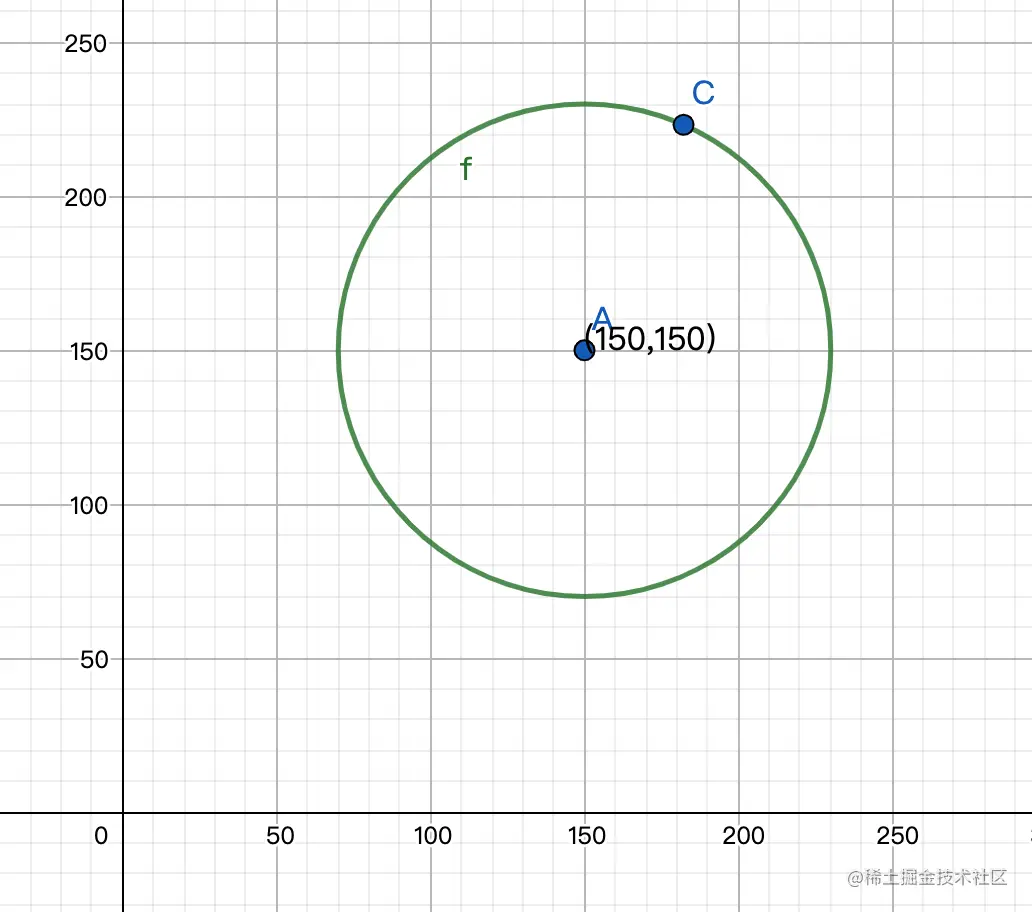// 计算要生成的多个粑粑的位置
// 传入的参数num为要生成的粑粑的数量
function randomPosition(num) {
const radius = 80; // 圆半径
const randomX = Math.random() * radius // 任取0到半径中的任意一个x
const y = Math.round(Math.sqrt(radius * radius - randomX * randomX)); // 确定一个第一象限在圆上的点
const radian = Math.atan(y / randomX); // 这个点的弧度值

const step = Math.PI * 2 / num; // 每坨屎间距的弧度值

return new Array(num).fill(0).map((item, index) => {
const r = (index * step + radian)
// 将弧度为0 - 2 * PI
const tr = r > Math.PI * 2 ? r - Math.PI * 2 : r < 0 ? r + Math.PI * 2 : r;
return {
}
})

}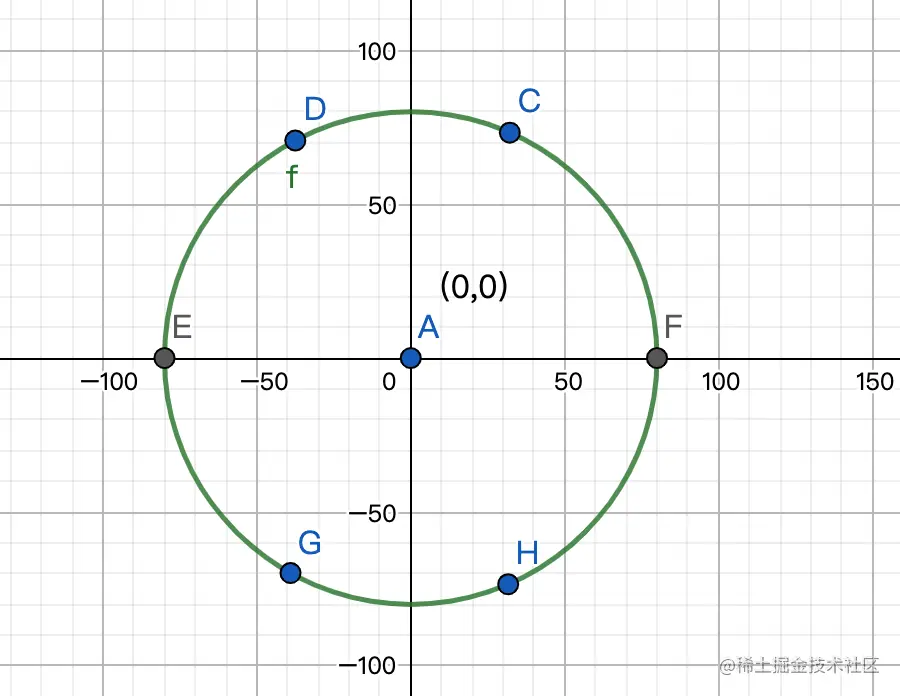randomPosition(6).map(item => ({ x: item.x + 150, y: item.y + 150 })) // 此处你也定义多于6个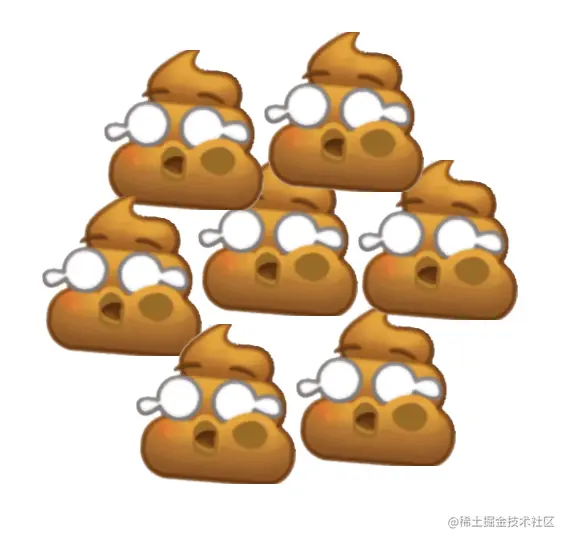const dis = Math.sqrt((end.x - 150) * (end.x - 150) + (end.y - 150) * (end.y - 150)); // 由于此时已经平移 150 ，因此需要计算距离中心点的距离
const r = Math.pow(2/3, dis / length); // 要缩放的比例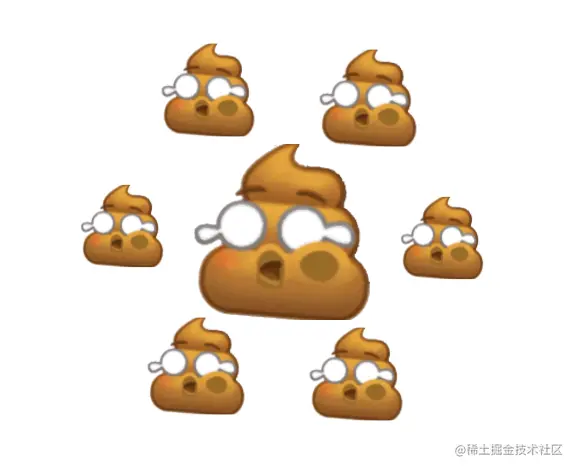function randomPosition(num) {
...
return new Array(num).fill(0).map((item, index) => {
const r = (index * step + radian)
const tr = r > Math.PI * 2 ? r - Math.PI * 2 : r < 0 ? r + Math.PI * 2 : r;
return {
// 增加随机值
x: length * Math.sin(tr) + (Math.random() > 0.5 ? 1 : -1) * 10 * Math.random(),
y: length * Math.cos(tr) + (Math.random() > 0.5 ? 1 : -1) * 10 * Math.random(),
}
})
}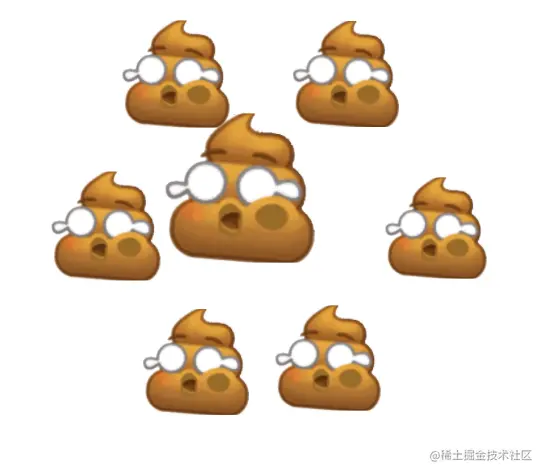#### 3.3角度

function createfeces(scale) {
const fece = document.createElement('div');
fece.className = 'feces';
const symbol = Math.random() > 0.5 ? 1 : -1; // 生成 -20 ~ 20 之间的随机角度
fece.style.transform = scale(${scale}) rotate(${symbol * 20 * Math.random()}deg)
fece.style.opacity = '0';
return fece;
}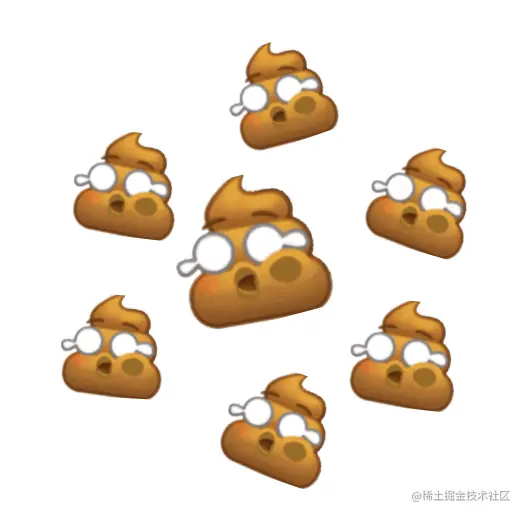#### 3.4动画

// 一开始的出现时候的动画，从爆炸口冲出来
function initFece(end) {
...
const start = { x: 0, y: 100, z: 0 }; // 爆炸口
const tween = new TWEEN.Tween(start)
.to({ ...end, z: 1 }, 100)
.easing(TWEEN.Easing.Linear.None)
.onUpdate(function () {
fece.style.setProperty('top', ${start.y}px); fece.style.setProperty('left', ${start.x}px);
fece.style.setProperty('opacity', ${start.z}); }) .onComplete(function () { initDown(start, fece).start(); // 冲出完成，进行下落透明动画 }) return tween; } // 下落同时变透明动画 function initDown(start, fece) { const s = { y: start.y, o: 1, }; const e = { y: start.y + 80, o: 0 }; const tween = new TWEEN.Tween(s) .to(e, 2000 + 500 * Math.random()) .easing(TWEEN.Easing.Quadratic.In) .onUpdate(function () { fece.style.setProperty('top', ${s.y}px);
fece.style.setProperty('opacity', \${s.o});
})
.onComplete(function () {
})
return tween;
}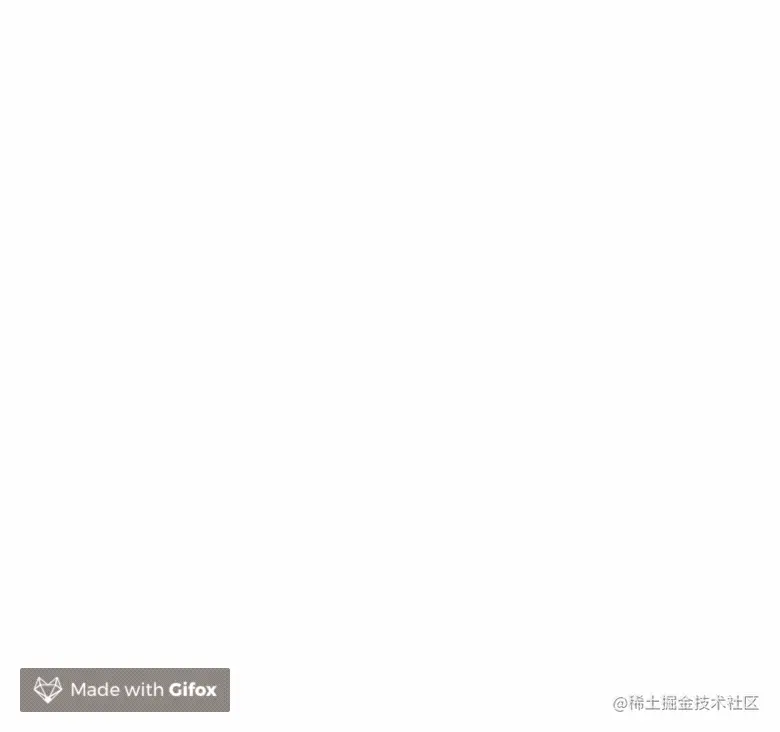#### 3.5总结

• 先利用 1 = x² + y² 圆轨迹的特性，建立初步位置
• 再通过加入随机值，使得整个分布稍微不那么规则一些
• 给粑粑添加随机角度
• 让中心粑粑更趋向于爆炸口
• 添加链式动画出现和下落

## 结尾

### 结语

❤️关注+点赞+收藏+评论+转发❤️，原创不易，鼓励笔者创作更好的文章